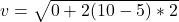## A spring gun with a 75 N/m spring constant is loaded with a 5 g foam dart and isaimed vertically. When the spring is compressed by 10 cm and

Question

A spring gun with a 75 N/m spring constant is loaded with a 5 g foam dart and isaimed vertically. When the spring is compressed by 10 cm and then released, the fireddart rises to a max height of 5 m above the end of the spring gun. Assuming the dartexperiences a constant friction force due to the air, how fast is it traveling when ithas fallen 2 m from its maximum height

in progress 0
6 months 2021-07-17T21:08:50+00:00 1 Answers 7 views 0

The speed is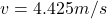Explanation:

From the question we are told that

The spring constant is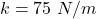The mass of the foam dart is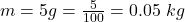The compression distance is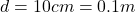The height which the gun raised the dart is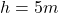The change in height is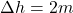The new height is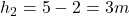Generally from the law of conservation of energy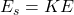Where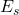is the energy stored in spring and it is mathematically represented as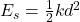KE is the kinetic energy possessed by the dart when it is being shut and this is mathematically represented as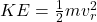So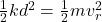Substituting values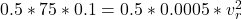=>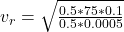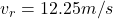When the dart is at  the maximum height the

let it acceleration due air resistance be z

So by equation of motion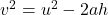Where v is the velocity at maximum height which is equal to zero

and  u is it initial velocity before reaching maximum height which we calculated asand a is the acceleration due to gravity + the acceleration due to air resistance

So

a =  z+g

=  9.8 + z

=>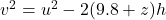Substituting values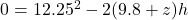Making z the subject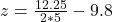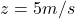When the dart is moving downward we can mathematically represent the motion as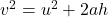Since the motion is downward and air resistance is upward we have that

a =  g – z

and the the initial velocity u becomes the velocity at maximum height

i.e u = 0

And v is the velocity the dart has when it is moving downward

So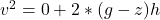Substituting values